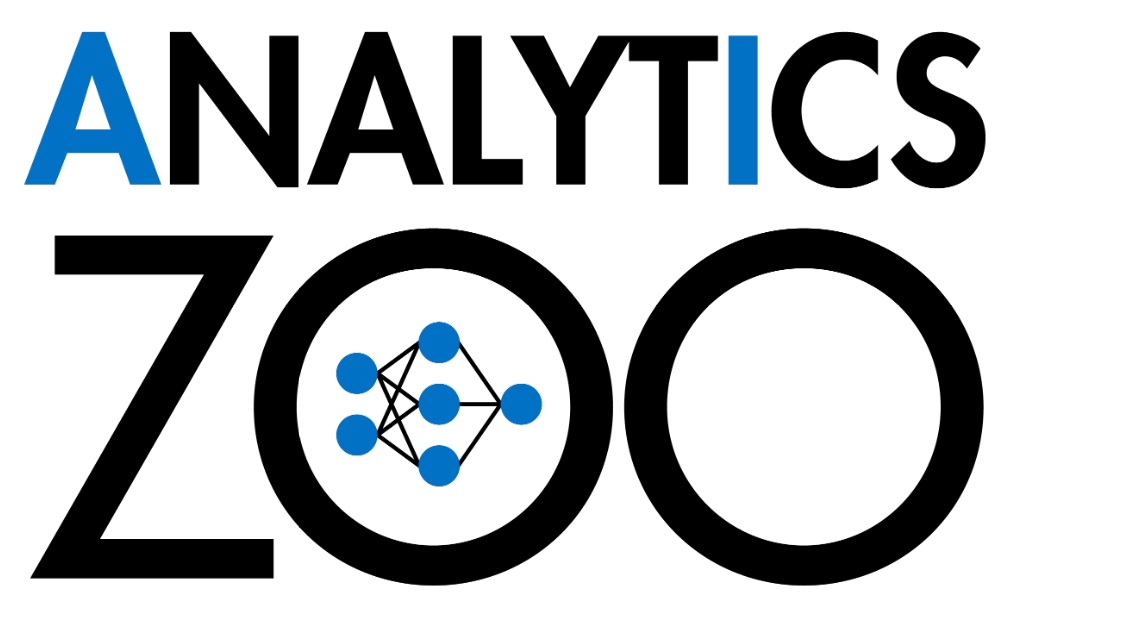Note: We are merging Analytics Zoo into BigDL 2.0, and our future development will move to the BigDL projectDistributed TensorFlow, PyTorch, Keras and BigDL on Apache Spark & Ray

Analytics Zoo is an open source Big Data AI platform, and includes the following features for scaling end-to-end AI to distributed Big Data:

• Orca: seamlessly scale out TensorFlow and PyTorch for Big Data (using Spark & Ray)

• RayOnSpark: run Ray programs directly on Big Data clusters

• BigDL Extensions: high-level Spark ML pipeline and Keras-like APIs for BigDL

• Chronos: scalable time series analysis using AutoML

• PPML: privacy preserving big data analysis and machine learning (experimental)

## Installing

You can use Analytics Zoo on Google Colab without any installation. Analytics Zoo also includes a set of notebooks that you can directly open and run in Colab.

To install Analytics Zoo, we recommend using conda environments.

``````conda create -n my_env
conda activate my_env
pip install analytics-zoo
``````

To install latest nightly build, use `pip install --pre --upgrade analytics-zoo`; see Python and Scala user guide for more details.

## Getting Started with Orca

Most AI projects start with a Python notebook running on a single laptop; however, one usually needs to go through a mountain of pains to scale it to handle larger data set in a distributed fashion. The Orca library seamlessly scales out your single node TensorFlow or PyTorch notebook across large clusters (so as to process distributed Big Data).

First, initialize Orca Context:

``````from zoo.orca import init_orca_context

# cluster_mode can be "local", "k8s" or "yarn"
sc = init_orca_context(cluster_mode="yarn", cores=4, memory="10g", num_nodes=2)
``````

Next, perform data-parallel processing in Orca (supporting standard Spark Dataframes, TensorFlow Dataset, PyTorch DataLoader, Pandas, etc.):

``````from pyspark.sql.functions import array

df = df.withColumn('user', array('user')) \
.withColumn('item', array('item'))
``````

Finally, use sklearn-style Estimator APIs in Orca to perform distributed TensorFlow, PyTorch or Keras training and inference:

``````from tensorflow import keras
from zoo.orca.learn.tf.estimator import Estimator

user = keras.layers.Input(shape=)
item = keras.layers.Input(shape=)
feat = keras.layers.concatenate([user, item], axis=1)
predictions = keras.layers.Dense(2, activation='softmax')(feat)
model = keras.models.Model(inputs=[user, item], outputs=predictions)
model.compile(optimizer='rmsprop',
loss='sparse_categorical_crossentropy',
metrics=['accuracy'])

est = Estimator.from_keras(keras_model=model)
est.fit(data=df,
batch_size=64,
epochs=4,
feature_cols=['user', 'item'],
label_cols=['label'])
``````

See TensorFlow and PyTorch quickstart, as well as the document website, for more details.

## Getting Started with RayOnSpark

Ray is an open source distributed framework for emerging AI applications. RayOnSpark allows users to directly run Ray programs on existing Big Data clusters, and directly write Ray code inline with their Spark code (so as to process the in-memory Spark RDDs or DataFrames).

``````from zoo.orca import init_orca_context

# cluster_mode can be "local", "k8s" or "yarn"
sc = init_orca_context(cluster_mode="yarn", cores=4, memory="10g", num_nodes=2, init_ray_on_spark=True)

import ray

@ray.remote
class Counter(object):
def __init__(self):
self.n = 0

def increment(self):
self.n += 1
return self.n

counters = [Counter.remote() for i in range(5)]
print(ray.get([c.increment.remote() for c in counters]))
``````

See the RayOnSpark user guide and quickstart for more details.

## Getting Started with BigDL Extensions

Analytics Zoo makes it easier to develop large-scale deep learning applications on Apache Spark, by providing high-level Spark ML pipeline and Keras-like APIs on top of BigDL (a distributed deep learning framework for Spark).

First, call `initNNContext` at the beginning of the code:

``````import com.intel.analytics.zoo.common.NNContext
val sc = NNContext.initNNContext()
``````

Then, define the BigDL model using Keras-style API:

``````val input = Input[Float](inputShape = Shape(10))
val dense = Dense[Float](12).inputs(input)
val output = Activation[Float]("softmax").inputs(dense)
val model = Model(input, output)
``````

After that, use `NNEstimator` to train/predict/evaluate the model using Spark Dataframes and ML pipelines:

``````val trainingDF = spark.read.parquet("train_data")
val scaler = new MinMaxScaler().setInputCol("in").setOutputCol("value")
val estimator = NNEstimator(model, CrossEntropyCriterion())
val pipeline = new Pipeline().setStages(Array(scaler, estimator))

val pipelineModel = pipeline.fit(trainingDF)
val predictions = pipelineModel.transform(validationDF)
``````

See the Scala, NNframes and Keras API user guides for more details.

## Getting Started with Chronos

Time series prediction takes observations from previous time steps as input and predicts the values at future time steps. The Chronos library makes it easy to build end-to-end time series analysis by applying AutoML to extremely large-scale time series prediction.

To train a time series model with AutoML, first initialize Orca Context:

``````from zoo.orca import init_orca_context

#cluster_mode can be "local", "k8s" or "yarn"
sc = init_orca_context(cluster_mode="yarn", cores=4, memory="10g", num_nodes=2, init_ray_on_spark=True)
``````

Next, create an AutoTSTrainer.

``````from zoo.chronos.autots.deprecated.forecast import AutoTSTrainer

trainer = AutoTSTrainer(dt_col="datetime", target_col="value")
``````

Finally, call `fit` on AutoTSTrainer, which applies AutoML to find the best model and hyper-parameters; it returns a TSPipeline which can be used for prediction or evaluation.

``````#train a pipeline with AutoML support
ts_pipeline = trainer.fit(train_df, validation_df)

#predict
ts_pipeline.predict(test_df)
``````

See the Chronos user guide and example for more details.

## PPML (Privacy Preserving Machine Learning)

Analytics Zoo PPML provides a Trusted Cluster Environment for protecting the end-to-end Big Data AI pipeline. It combines various low level hardware and software security technologies (e.g., Intel SGX, LibOS such as Graphene and Occlum, Federated Learning, etc.), and allows users to run unmodified Big Data analysis and ML/DL programs (such as Apache Spark, Apache Flink, Tensorflow, PyTorch, etc.) in a secure fashion on (private or public) cloud.

See the PPML user guide for more details.

Older Documents

Alternatives To Analytics Zoo
Select To Compare

Alternative Project Comparisons
Related Awesome Lists

Get A Weekly Email With Trending Projects For These Topics
No Spam. Unsubscribe easily at any time.
Python (809,126
Jupyter Notebook (154,800
Scala (28,681
Tensorflow (22,362
Pytorch (21,088
Spark (10,735
Keras (10,453
Deep Neural Networks (3,913
Ray (3,760
Apache Spark (1,194